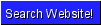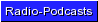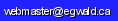Egwald Economics: Microeconomics

Duality and the Cobb-Douglas Production / Cost Functions

by

Elmer G. Wiens

Egwald's popular web pages are provided without cost to users.
Please show your support by joining Egwald Web Services as a Facebook Fan:Follow Elmer Wiens on Twitter:Duality: Production / Cost Functions:   Cobb-Douglas Duality | CES Duality | Theory of Duality | Translog Duality - CES | Translog Duality - Generalized CES | References and Links

O. Duality and the Cobb-Douglas Production / Cost Functions.

Production and cost functions (and profit functions) can be used to model how a profit (wealth) maximizing firm hires or purchases inputs (factors), such as labour, capital (structures and machinery), and materials and supplies, and combines these inputs through its production process to produce the products (outputs) that the firm sells (supplies) to its customers. The theory of duality links the production function models to the cost function models by way of a minimization or maximization framework. The cost function is derived from the production function by choosing the combination of factor quantities that minimize the cost of producing levels of output at given factor prices. Conversely, the production function is derived from the cost function by calculating the maximum level of output that can be obtained from specified combinations of inputs.

I. Cobb-Douglas Production Function.

A production function is a technological relationship between the levels of output that can be produced using specific combinations of factor inputs.

The three factor Cobb-Douglas production function is:

q = A * (L^alpha) * (K^beta) * (M^gamma) = f(L,K,M).

where L = labour, K = capital, M = materials and supplies, and q = product. The coefficients of the production function, A, alpha, beta, and gamma are positive, real numbers. The production function's inputs, L, K, and M, are non-negative real numbers.

II. Cobb-Douglas Cost Function.

A cost function is a relationship between the total cost of producing levels of output, q, at specific values of factor input prices, wL, wK, and wM, using the cost minimizing combinations of factor inputs.

The three factor Cobb-Douglas cost function is:

C(q;wL,wK,wM) = h(q) * c(wL,wK,wM)

where the returns to scale function is:

h(q) = q^(1/(alpha+beta+gamma))

a continuous, increasing function of q (q >= 1), with h(0) = 0 and h(1) = 1.

and the unit cost function is:

c(wL,wK,wM) = B * [ wL^alpha * wK^beta * wM^gamma] ^(1/(alpha+beta+gamma))

with B = (alpha + beta + gamma) / [A * alpha^alpha * beta^beta * gamma^gamma] ^(1/(alpha+beta+gamma))

III. Cobb-Douglas Production Function Partial Derivatives.

 fL(L,K,M) = A * alpha * (L^alpha-1) * (K^beta) * (M^gamma),   → fL(L,K,M) = (alpha / L) * f(L,K,M); so similarly fK(L,K,M) = (beta / K) * f(L,K,M); fM(L,K,M) = (gamma / M) * f(L,K,M).

IV. Cobb-Douglas Cost Function Partial Derivatives.

 ∂C(q;wL,wK,wM) / ∂wL = h(q) * (alpha / L) * c(wL, wK, wM) / (alpha + beta + gamma) ; so similarly ∂C(q;wL,wK,wM) / ∂wK = h(q) * (beta / K) * c(wL, wK, wM) / (alpha + beta + gamma); ∂C(q;wL,wK,wM) / ∂wM = h(q) * (gamma / M) * c(wL, wK, wM) / (alpha + beta + gamma).

V. Duality: Production to Cost Function.

Find the values of L, K, M, and µ that minimize the Lagrangian:

G(q;L,K,M,µ) = wL * L + wK * K + wM* M + µ * [q - f(L,K,M)]

 GL = wL - µ * fL = 0 GK = wK - µ * fK = 0 GM = wM - µ * fM = 0 Gµ = q - f(L,K,M) = 0

From equations a., b., and c. we get:

 e.   wL / wK = fL / fK = alpha * L / (beta * K) --> K = L * beta * wL / (alpha * wK) f.   wL / wM = fL / fM = alpha * L / (gamma * M) --> M = L * gamma * wL / (alpha * wM) g.   wK / wM = fK / fM = beta * K / (gamma * M)

Substituting equations e. and f. into the Cobb-Douglas production function and solving for L yields;

 L = {q / [A* (beta*wL/(alpha*wK))^beta * (gamma*wL/(alpha*wM))^gamma]}^(1/(alpha+beta+gamma))     = q(1/(alpha+beta+gamma)) * (alpha / wL) * [ wL^alpha * wK^beta * wM^gamma/ (A * alpha^alpha * beta^beta * gamma^gamma)] ^(1/(alpha+beta+gamma))

Finally, substituting e., f. and h. into the cost function:

C(q;wL,wK,wM) = wL * L + wk * K + wM * M

yields the cost function, as a function of output, depending on the input prices and the parameters of the Cobb-Douglas production function.

To see this write:

R = wL^alpha * wK^beta * wM^gamma/ (A * alpha^alpha * beta^beta * gamma^gamma), and
B = (alpha+beta+gamma) / [A * alpha^alpha * beta^beta * gamma^gamma]^(1/(alpha+beta+gamma))

Then:

 i.   L = q^1/(alpha+beta+gamma) * (alpha / wL) * R^1/(alpha+beta+gamma), j.   K = q^1/(alpha+beta+gamma) * (beta / wK) * R^1/(alpha+beta+gamma), k.   M = q^1/(alpha+beta+gamma) * (gamma / wM) * R^1/(alpha+beta+gamma).

Therefore:

C(q;wL,wK,wM) = wL*q^1/(alpha+beta+gamma)*(alpha / wL)*R^1/(alpha+beta+gamma) + wK*q^1/(alpha+beta+gamma)*(beta / wK)*R^1/(alpha+beta+gamma) + wM*(q^1/(alpha+beta+gamma)*(gamma / wM)*R^1/(alpha+beta+gamma),  →

C(q;wL,wK,wM) = q^1/(alpha+beta+gamma) * (alpha+beta+gamma) * R^1/(alpha+beta+gamma),  →

C(q;wL,wK,wM) = h(q) * B * [wL^alpha * wK^beta * wM^gamma]^1/(alpha+beta+gamma) = h(q) * c(wL,wK,wM).

VI. Cobb-Douglas Factor Demand Functions (Cost Minimizing Factor Inputs).

If we take the derivative of the cost function with respect to an input price, we get the factor demand function for that input:

 l.   ∂C(q;wL,wK,wM) / ∂wL   =   L(q;wL,wK,wM)   =   h(q) * l(wL,wK,wM)   = h(q) * (alpha / wL) * c(wL,wK,wM) / (alpha + beta + gamma) m.   ∂C(q;wL,wK,wM) / ∂wK   =   K(q;wL,wK,wM)   =   h(q) * m(wL,wK,wM)   = h(q) * (beta / wK) * c(wL,wK,wM) / (alpha + beta + gamma) n.   ∂C(q;wL,wK,wM) / ∂wM   =   M(q;wL,wK,wM)   =   h(q) * m(wL,wK,wM)   = h(q) * (gamma / wM) * c(wL,wK,wM) / (alpha + beta + gamma)

Note: equations i. = l.,   j. = m.,   and k. = n.

VII. Duality: Cost to Production Function.

 l.   →   o.   wL = alpha * h(q) * c(wL,wK,wM) / [(alpha + beta + gamma) * L(q;wL,wK,wM)] m.   →   p.   wK = beta * h(q) * c(wL,wK,wM) / [(alpha + beta + gamma) * K(q;wL,wK,wM)] n.   →   q.   wM = gamma * h(q) * c(wL,wK,wM) / [(alpha + beta + gamma) * M(q;wL,wK,wM)]

Substituting equations o., p., and q. into the Cobb-Douglas unit cost function yields:

c(wL,wK,wM)   = B* { [alpha * h(q) * c(wL,wK,wM) / [(alpha+beta+gamma) * L(q;wL,wK,wM)]^alpha * [beta * h(q) * c(wL,wK,wM) / [(alpha+beta+gamma) * K(q;wL,wK,wM)]^beta * [ gamma * h(q) * c(wL,wK,wM) / [(alpha+beta+gamma) * M(q;wL,wK,wM)]^gamma }^1/(alpha+beta+gamma)

After factoring [h(q) * c(wL,wK,wM) / (alpha+beta+gamma)]^(alpha+beta+gamma):

c(wL,wK,wM)   =   [h(q) * c(wL,wK,wM) / (alpha+beta+gamma)] * (alpha + beta + gamma) / [A * alpha^alpha * beta^beta * gamma^gamma] ^(1/(alpha+beta+gamma)) * { alpha^alpha * beta^beta * gamma^gamma / [ (L^alpha) * (K^beta) * (M^gamma) ] }^1/(alpha+beta+gamma),

c(wL,wK,wM)   =   h(q) * c(wL,wK,wM) / [ A * (L^alpha) * (K^beta) * (M^gamma)] ^1/(alpha+beta+gamma).

Cancelling c(wL,wK,wM) and solving for h(q):

h(q)   =   [ A * (L^alpha) * (K^beta) * (M^gamma)]^1/(alpha+beta+gamma).

Using the definition of h(q) = q^1/(alpha+beta+gamma):

q   =   A * (L^alpha) * (K^beta) * (M^gamma) = f(L,K,M).

VIII. Properties of the Cobb-Douglas Production Function, f(L,K,M).

a. If all factor inputs are zero, the level of product output is zero.

f(0, 0, 0) = 0.

b. Any increase in factor inputs will NOT decrease the level of product output.

L1 <= L2, K1 <= K2, and M1 <= M2   →   f(L1, K1, M1) <= f(L2, K2, M2).

c. The Cobb-Douglas production function's elasticity of scale is determined by the parameter nu = (alpha + beta + gamma):

nu < 1   →   decreasing returns to scale;   nu = 1   →   constant returns to scale;   nu > 1   →   increasing returns to scale.

d. The Cobb-Douglas production function, f, is continuous and twice-continuously differentiable for L > 0, K > 0, and M > 0.

e. The Cobb-Douglas production function, f, is is concave to the origin of the 3-dimensional (L,K, M) space for L > 0, K > 0, and M > 0.

f. The Cobb-Douglas production function can also be written as:

q = A * L^alpha * K^beta * M^gamma = A * [L^alpha1 * K^beta1 * M^gamma1]^nu,

where nu = (alpha + beta + gamma), alpha1 = alpha / nu, beta1 = beta / nu, and gamma1 = gamma / nu   →   (alpha1 + beta1 + gamma1) = 1.

IX. Properties of the Unit Cobb-Douglas Cost Function, c(wL,wK,wM).

a. c is linear homogeneous in factor prices.

c(t*wL, t*wK, t*wM) = B*[(t*wL)^alpha * (t*wK)^beta * (t*wM)^gamma]^(1/(alpha+beta+gamma))

= t * c(wL, wK, wM)

b. The matrix ∇2c of second order partial derivatives of the unit cost function c(wL,wK,wM) is symmetric.

 ∂(∂c(wL,wK,wM)/∂wL)/∂wK = ∂([(alpha / wL) * c(wL,wK,wM)])/∂wK = alpha * beta / (wL * wK) * c(wL,wK,wM) = ∂(∂c(wL,wK,wM)/∂wK)/∂wL ∂(∂c(wL,wK,wM)/∂wL)/∂wM = ∂([(alpha / wL) * c(wL,wK,wM)]))/∂wM = alpha * gamma / (wL * wM) * c(wL,wK,wM) = ∂(∂c(wL,wK,wM)/∂wM)/∂wL etc.

c. The unit cost function c is concave in factor prices.

d. The Cobb-Douglas production function is called homothetic, because the Cobb-Douglas cost function can be separated (factored) into a function of output, q, times a function of input prices, wL, wK, and wM.

e. The marginal cost function can be obtained by differentiating the Cobb-Douglas cost function with respect to output q:

C(q;wL,wK,wM) = h(q) * c(wL,wK,wM)   →
MC(q;wL,wK,wM) = ∂(C(q;wL,wK,wM))/ ∂q = h'(q) * c(wL,wK,wM) = (1/(alpha+beta+gamma)) * q ^(1 - (alpha+beta+gamma))/(alpha+beta+gamma) * c(wL,wK,wM)

f. The supply function, for competitive product market conditions, can be obtained by setting marginal cost to P, the price of the output:

P = MC(q;wL,wK,wM) = h'(q) * c(wL,wK,wM) = (1/(alpha+beta+gamma)) * q ^(1 - (alpha+beta+gamma))/(alpha+beta+gamma) * c(wL,wK,wM)   →
q = [(alpha+beta+gamma) * P / c(wL,wK,wM)]^(alpha+beta+gamma)/(1 - (alpha+beta+gamma))

X. Elasticity of substitution between inputs (sigma).

From equation e. of Part V we get:

K/L = (beta / alpha) * (wl / wK) → ln(K/L) = ln(beta/alpha) + ln(wL/wK)

sigma = d(ln(K/L))/d(ln(wL/wK)) = 1

XI. Negative definite:

The Hessian, H, of a function, f is negative definite, if the principal minors of H alternate in sign, starting with negative. If one (or more) principal minors have a zero value, f is negative semidefinite.

For the Cobb-Douglas production function:

 H = fLL fLK fLM fKL fKK fKM fML fMK fMM

H1 = fLL = -alpha * f * (1 - alpha) / L^2 < 0

 H2 = fLL fLK fKL fKK

 H2 = -alpha*f*(1 - alpha)/L^2 alpha*beta*f/(L*K) alpha*beta*f/(L*K) -beta * f * (1 - beta)/ K^2

H2 = [alpha*beta/(L^2*K^2)] * f * (1 - alpha - beta) > 0

H3 = determinate of H <(=) 0

XII. Numerical examples:

The web page, "The Duality of Production and Cost Functions," permits one to specify the parameters of the Cobb-Douglas (or CES) production function, and to ascertain the curvature of the production function and corresponding cost function.Copyright © Elmer G. Wiens:   Egwald Web ServicesAll Rights Reserved.    Inquiries